# DCF Model Training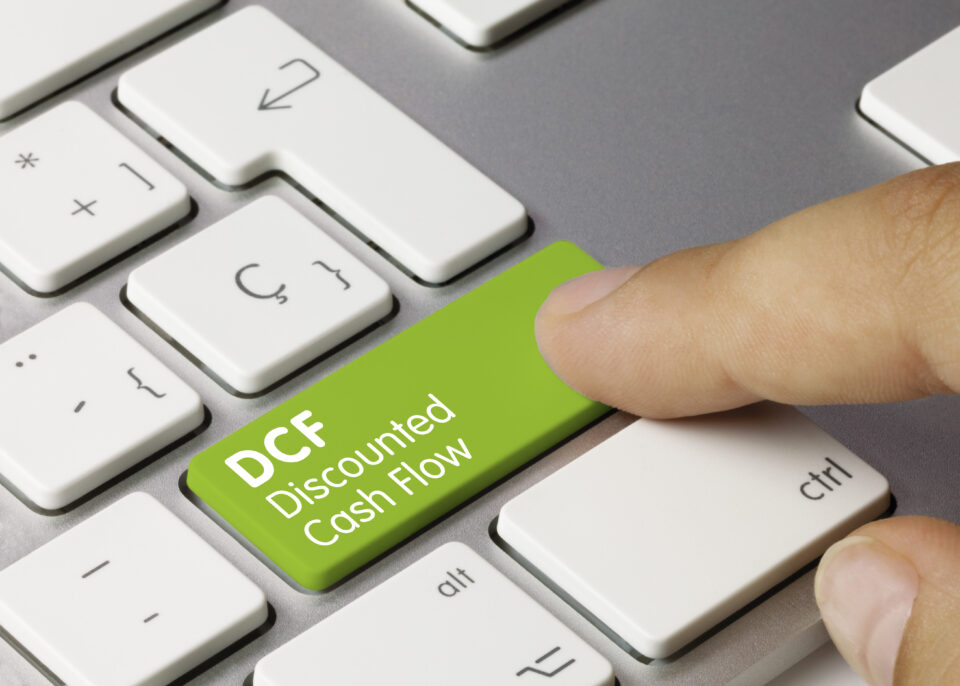## Who this DCF guide is for

We wrote this guide for those thinking about a career in finance and those in the early stages of preparing for job interviews. This guide is quite detailed but it stops short of all corner cases and nuances of a fully fledged DCF model. For that, you can enroll in our full scale modeling course.

## DCF basics: The present value formula

The DCF approach requires that we forecast a company’s cash flows into the future and discount them to the present in order to arrive at a present value for the company. That present value is the amount investors should be willing to pay (the company’s value). We can express this formulaically as (we denote the discount rate as r):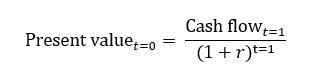So, let’s say you decide you’re willing to pay \$800. We can solve this as: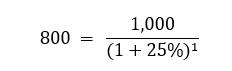If I make the same proposition but instead of only promising \$1,000 next year, say I promise \$1,000 for the next 5 years. The math gets only slightly more complicated: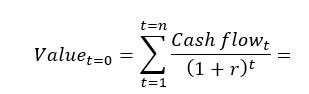In Excel, you can calculate this fairly easily using the PV function (see below). However, if cash flows are different each year, you will have to discount each cash flow separately: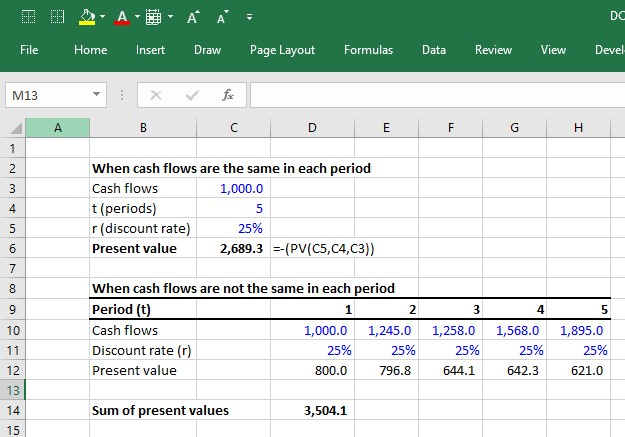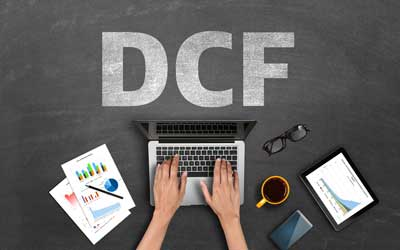Submitting ...

## 6 steps to building a DCF

The premise of the DCF model is that the value of a business is purely a function of its future cash flows. Thus, the first challenge in building a DCF model is to define and calculate the cash flows that a business generates. There are two common approaches to calculating the cash flows that a business generates.

1. Unlevered DCF approach
Forecast and discount the operating cash flows. Then, when you have a present value, just add any non-operating assets such as cash and subtract any financing related liabilities such as debt.
2. Levered DCF approach
Forecast and discount the cash flows that remain available to equity shareholders after cash flows to all non-equity claims (i.e. debt) have been removed.

Both should theoretically lead to the same value at the end (though in practice it’s actually pretty hard to get them to exactly equal). The unlevered DCF approach is the most common and is thus the focus of this guide. This approach involves 6 steps:

#### 1. Forecasting unlevered free cash flows

Step 1 is to forecast the cash flows a company generates from its core operations after accounting for all operating expenses and investments. These cash flows are called “unlevered free cash flows.”

#### 2. Calculating terminal value

You can’t keep forecasting cash flows forever. At some point, you must make some high level assumptions about cash flows beyond the final explicit forecast year by estimating a lump-sum value of the business past its explicit forecast period. That lump sum is called the “terminal value.”

#### 3. Discounting the cash flows to the present at the weighted average cost of capital

The discount rate that reflects the riskiness of the unlevered free cash flows is called the weighted average cost of capital. Because unlevered free cash flows represent all operating cash flows, these cash flows “belong” to both the company’s lenders and owners. As such, the risks of both providers of capital need to be accounted for using appropriate capital structure weights (hence the term “weighted average” cost of capital). Once discounted, the present value of all unlevered free cash flows is called the enterprise value.

#### 4. Add the value of non-operating assets to the present value of unlevered free cash flows

If a company has any non-operating assets such as cash or has some investments just sitting on the balance sheet, we must add them to the present value of unlevered free cash flows. For example, if we calculate that the present value of Apple’s unlevered free cash flows is \$700 billion, but then we discover that Apple also has \$200 billion in cash just sitting around, we should add this cash.

#### 5. Subtract debt and other non-equity claims

The ultimate goal of the DCF is to get at what belongs to the equity owners (equity value). Therefore if a company has any lenders (or any other non-equity claims against the business), we need to subtract this from the present value. What’s left over belongs to the equity owners.

In our example, if Apple had \$50 billion in debt obligations at the valuation date, the equity value would be calculated as:

\$700 billion (enterprise value) + \$200 billion (non-operating assets) – \$50 (debt) = \$850 billion

Often, the non-operating assets and debt claims are added together as one term called net debt (debt and other non-equity claims – non-operating assets). You’ll often see the equation: enterprise value – net debt = equity value. The equity value that the DCF spits out can now be compared to the market capitalization (that’s the market’s perception of the equity value).

#### 6. Divide the equity value by the shares outstanding

The equity value tells us what the total value to owners is. But what is the value of each share? In order to calculate this, we divide the equity value by the company’s diluted shares outstanding.

Now let’s break down each step into more detail.

## Calculating the unlevered free cash flows (FCF)

Here is the unlevered free cash flow formula:

FCF = EBIT x (1- tax rate) + D&A + NWC – Capital expenditures

• EBIT = Earnings before interest and taxes. This represents a company’s GAAP-based operating profit.
• Tax rate = The tax rate the company is expected to face. When forecasting taxes, we usually use a company’s historical effective tax rate.
• D&A = depreciation and amortization.
• NWC = Annual changes in net working capital. Increases in NWC are cash outflows while decreases are cash inflows.
• Capital expenditures represent cash investments the company must make in order to sustain the forecast growth of the business. If you don’t factor in the cost of required reinvestment into the business, you will overstate the value of the company by giving it credit for EBIT growth without accounting for the investments required to achieve it.

### FCFs are ideally driven from a 3-statement model

Forecasting all these line items should ideally come from a 3-statement model because all of the components of unlevered free cash flows are interrelated; Changes in EBIT assumptions impact capex, NWC and D&A. Without a 3-statement model that dynamically links all these together, it is difficult to ensure that changes in assumptions of one component correctly impact other components.

Because this takes more work and more time, finance professionals often do preliminary analyses using a quick, back-of-the-envelope DCF model and only build a full DCF model driven by a 3-statement model when the stakes are high, such as when an investment banking deal goes “live” or when a private equity firm is in the later stages of the investment process.

### The 2-stage DCF model

The 3-statement models that support a DCF are usually annual models that forecast about 5-10 years into the future. However, when valuing businesses we usually assume they are a going concern.  In other words, they will continue to operate forever.

That means that the 3-statement model only takes us so far. We also have to forecast the present value of all future unlevered free cash flows after the explicit forecast period. This is called the 2-stage DCF model. The first stage is to forecast the unlevered free cash flows explicitly (and ideally from a 3-statement model). The second stage is the total of all cash flows after stage 1. This typically entails making some assumptions about the company reaching mature growth. The present value of the stage 2 cash flows is called the terminal value.

Prefer video? To watch a free video lesson on how to build a DCF, click here

### Calculating the terminal value

In a DCF, the terminal value (TV) represents the value the company will generate from all the expected free cash flows after the explicit forecast period. Imagine that we calculate the following unlevered free cash flows for Apple: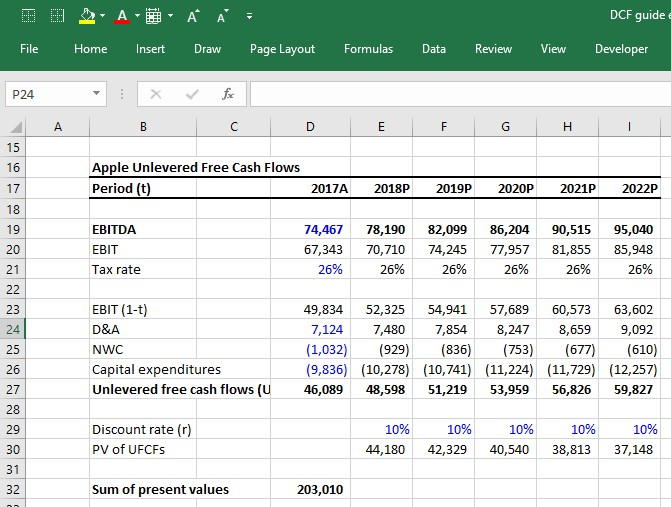Apple is expected to generate cash flows beyond 2022, but we cannot project FCFs forever (with any degree of accuracy). So how do we estimate the value of Apple beyond 2022? There are two prevailing approaches:

1. Growth in perpetuity
2. Exit EBITDA multiple method

#### The growth in perpetuity approach

The growth in perpetuity approach assumes Apple’s UFCFs will grow at some constant growth rate assumption from 2022 to … forever. The formula for calculating the present value of a cash flow growing at a constant growth rate in perpetuity is called the “Growth in perpetuity formula.” It is: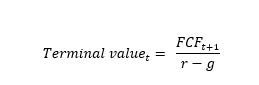If we assume that after 2022, Apple’s UFCFs will grow at a constant 4% rate in perpetuity and will face a weighted average cost of capital of 10% in perpetuity, the terminal value (which is the present value of all Apple’s future cash flows beyond 2022) is calculated as: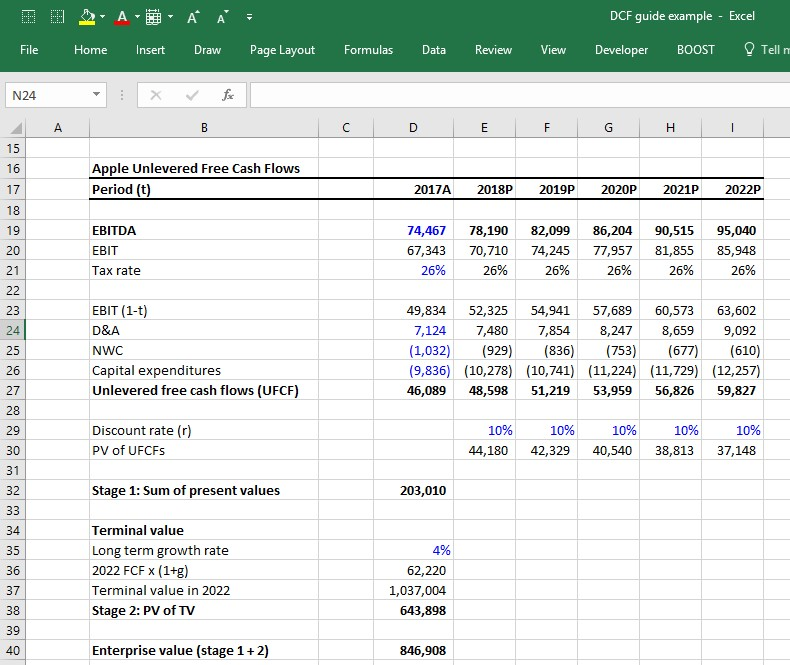At this point, notice that we have finally calculated enterprise value as simply the sum of the stage 1 present value of UFCFs + the present value of stage 2 terminal value.

#### Exit EBITDA multiple method

The growth in perpetuity approach forces us to take a guess as to the long-term growth rate of a company. The result of the analysis is very sensitive to this assumption. A way around having to guess a company’s long term growth rate is to guess the EBITDA multiple the company will be valued at the last year of the stage 1 forecast.

A common way to do this is to look at the current EV/EBITDA multiple the company is trading at (or the average EV/EBITDA multiple of the company’s peer group) and assume the company will be valued at that same multiple in the future. For example, if Apple is currently valued at 9.0x its last twelve months (LTM) EBITDA, assume that in 2022 it will be valued at 9.0x its 2022 EBITDA.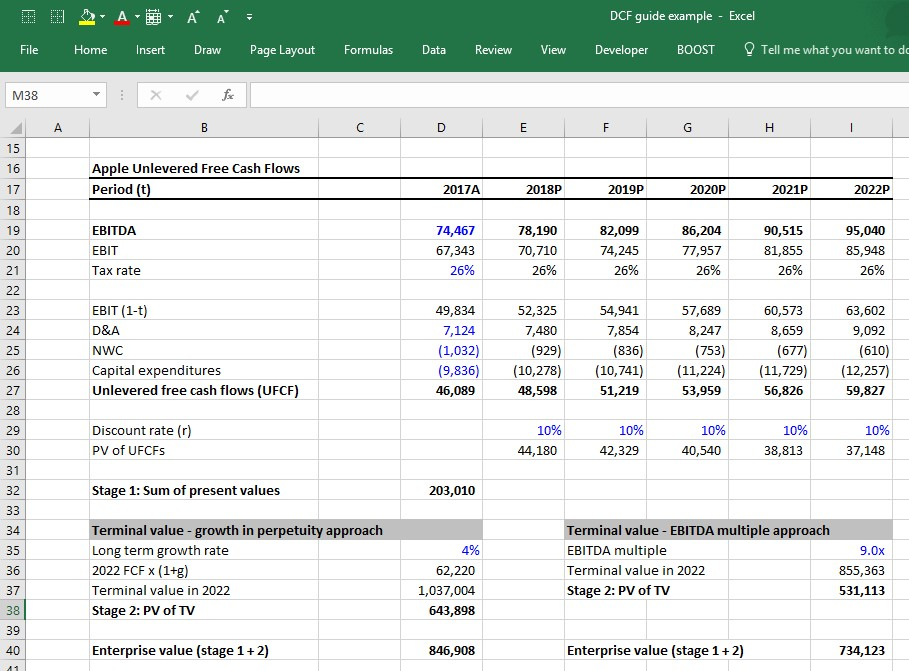#### Growth in perpetuity vs. exit EBITDA multiple method

Investment bankers and private equity professionals tend to be more comfortable with the EBITDA multiple approach because it infuses market reality into the DCF. A private equity professional building a DCF will likely try to figure out what he/she can sell the company for 5 years down the road, so this arguably provides a valuation that factors the EBITDA multiple in.

However, this approach suffers from a significant conceptual problem: It uses current market valuations in the DCF, which arguably defeats the whole purpose of the DCF. Making matters worse is the fact that the terminal value often represents a significant pecentage of the value contribution in a DCF, so the assumptions that go into calculating the terminal value are all the more important.Step-by-Step Online Course

#### Everything You Need To Master Financial Modeling

Enroll in The Premium Package: Learn Financial Statement Modeling, DCF, M&A, LBO and Comps. The same training program used at top investment banks.

### Getting to enterprise value: Discounting the cash flows by the WACC

Up to now, we’ve been assuming a 10% discount rate for Apple, but how is that quantified? Quantifying the discount rate, which in this case is the weighted average cost of capital (WACC), is a critical field of study in corporate finance. You can spend an entire college semester learning about it. We’ve written a complete guide to WACC here, but we’ll summarize the basic elements for how it is calculated:

The WACC formula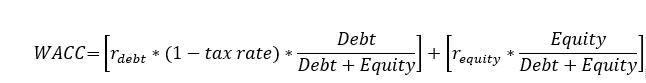Where:

### Getting to equity value: Adding the value of non-operating assets

Many companies have assets not directly tied to operations. Assets such as cash obviously increase the value of the company (i.e. a company whose operations are worth \$1 billion but also has \$100 million in cash is worth \$1.1 billion). But up to now, the value is not accounted for in the unlevered free cash flow calculation. Therefore, these assets need to be added to the value. The most common non-operating assets include:

Below is Apple 2016 year ending balance sheet. The non-operating assets are its cash and equivalents, short-term marketable securities and long-term marketable securities. As you can see, they represent a significant portion of the company’s balance sheet.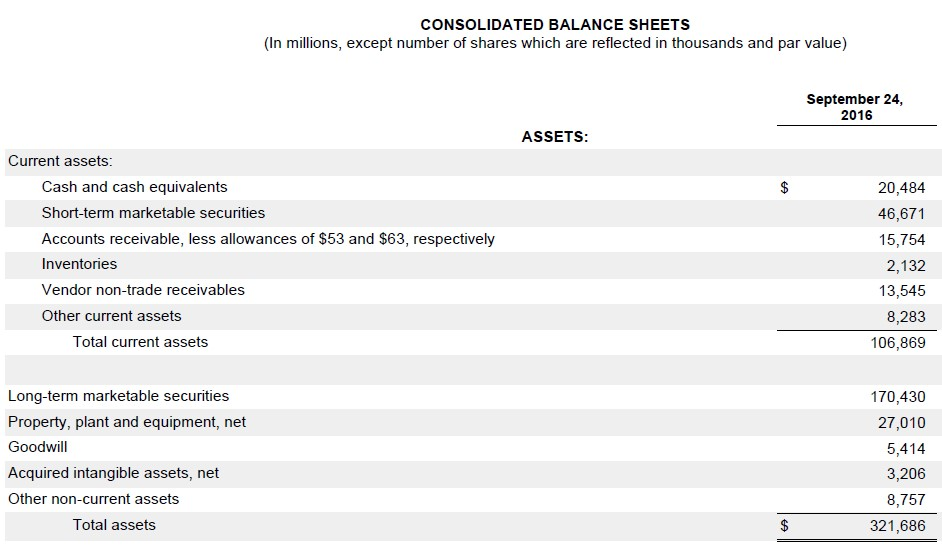Unlike operating assets such as PP&E, inventory and intangible assets, the carrying value of non-operating assets on the balance sheet is usually fairly close to their actual value. That’s because they are mostly comprised of cash and liquid investments that companies generally can mark up to fair value. That’s not always the case (equity investment is a notable exception), but it’s typically safe to simply use the latest balance sheet values of non-operating assets as the actual market values.

### Getting to equity value: Subtracting debt and other non-equity claims

At this point, we need to identify and subtract all non-equity claims on the business in order to arrive at how much of the company value actually belongs to equity owners. The most common non-equity claims you’ll encounter are:

• All debt (short term, long term, bonds, loans, etc..)
• Capital Leases
• Preferred stock
• Non-controlling (minority) interests

Below is Apple’s 2016 year ending balance sheet liabilities. You can see it has commercial paper, current portion of long term debt and long term debt. These are the three items that would make up Apple’s non-equity claims.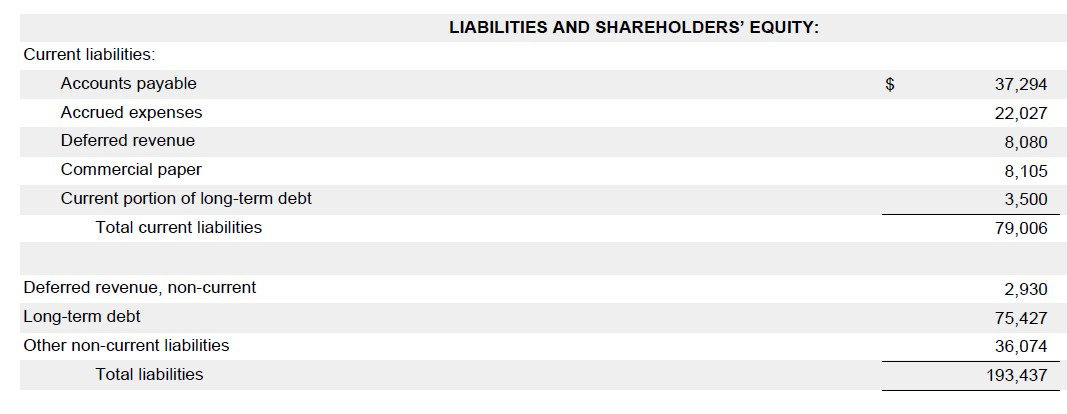As with the non-operating assets, finance professionals usually just use the latest balance sheet values of these items as a proxy for the actual values. This is usually a safe approach when the market values are fairly close to the balance sheet value.vThe market value of debt doesn’t usually deviate too much from the book value unless market interest rates have changed dramatically since the issue, or if the company’s credit profile has changed dramatically (i.e. a company in financial distress will have its debt trading at pennies on the dollar).

One place where the book value-as-proxy-for-market-value can be dangerous is “non-controlling interests.” Non-controlling interests are usually understated on the balance sheet. If they are significant, it is preferable to apply an industry multiple to better reflect their true value.

The bad news is that we rarely have enough insight into the nature of the non-controlling interests’ operations to figure out the right multiple to use. The good news is that non-controlling interests are rarely large enough to make a significant difference in valuation (most companies don’t have any).

Net debt formula

When building a DCF model, finance professionals often net non-operating assets against non-equity claims and call it net debt, which is subtracted from enterprise value to arrive at equity value, such that:

Enterprise value – net debt = Equity value

The formula for net debt is simply the value of all nonequity claims less the value of all non-operating assets:

Gross Debt (short term, long term, bonds, loans, etc..)

+ Capital Leases

+ Preferred stock

+ Non-controlling (minority) interests

– Cash

– Marketable securities

– Equity investments

Net debt

Using Apple’s 2016 10K, we can see that it has a substantial negative net debt balance. For companies that carry significant debt, a positive net debt balance is more common, while a negative net debt balance is common for companies that keep a lot of cash.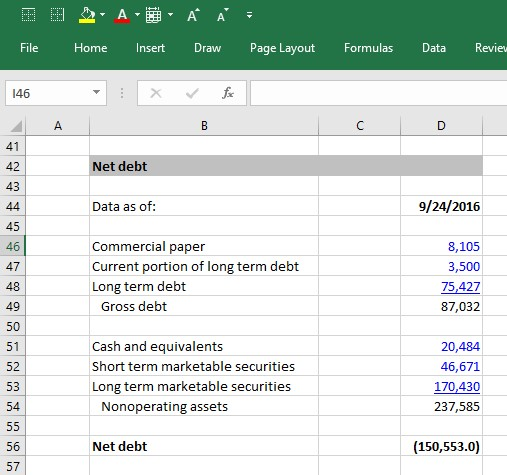### From equity value to equity value per share

Once a company’s equity value has been calculated, the next step is to determine the value of each individual share. In order to figure this out, we have to determine the number of shares that are currently outstanding. We have written a thorough guide to calculating a company’s current shares but will summarize here:

1. Take the current actual share count from the front cover of the company’s latest annual (10K) or interim (10Q) filing.  For Apple, it is: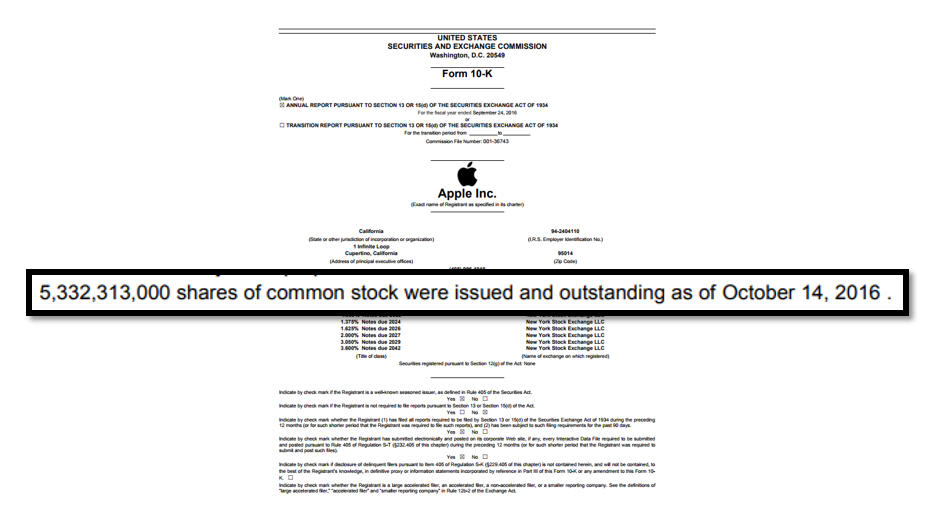2. Next, add the effect of dilutive shares. These are shares that aren’t quite common stock yet, but that can become common stock and thus be potentially dilutive to the common shareholders (i.e. stock options, warrants, restricted stock and convertible debt and convertible preferred stock).

Assuming that we calculated 50 million dilutive securities for Apple, we can now put all the pieces together and complete the analysis: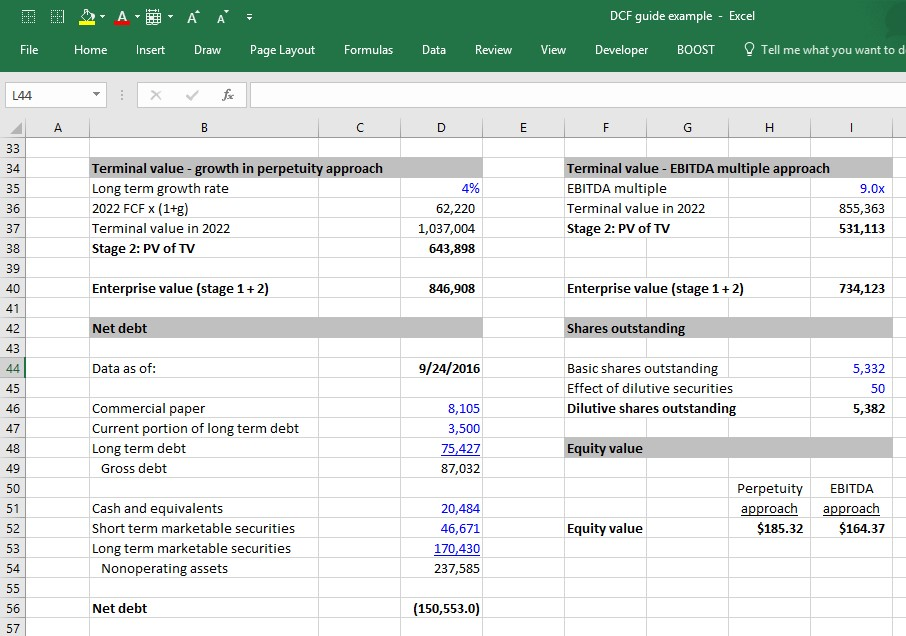## Three key assumptions in the DCF

We have now completed the 6 steps to building a DCF model and have calculated the equity value of Apple. What were the key assumptions that led us to the value we arrived at? The three key assumptions in a DCF model are:

1. The operating assumptions (revenue growth and operating margins)
2. The WACC
3. Terminal value assumptions: Long term growth rate and the exit multiple

Each of these assumptions is critical to getting an accurate model. In fact, the DCF model’s sensitivity to these assumptions, and the lack of confidence finance professionals have in these assumptions, (especially the WACC and terminal value) is frequently cited as the main weaknesses of the DCF model.

Nonetheless, the DCF model is one of the most common models used by investment bankers and other finance professionals, and the DCF output is almost always presented using a range of terminal value and WACC assumptions, as well as in context to other valuation methodologies. A common way this is presented is using a football field valuation matrix.

Prefer video? To watch a free video lesson on how to build a DCF, click here

## Enroll in Wall Street Prep’s complete DCF Modeling training

I hope you enjoyed this guide, which is a simplified version of our leading financial modeling training program. Our full program is used by the largest investment banks in the world to train new hires and by top business schools to prepare their students for careers in finance.

The full program is also available to individuals through our Premium Package self-study training program. You’ll learn how to build a comprehensive DCF model including  a variety of enhancements that can be added to improve a DCF model:

• Connecting a DCF model to a 3-statement model
• Stub year calculation
• Midyear discounting
• Using the DCF model to value an acquisition target
• Valuing synergies and NOLs in a DCF
• Calculating the cost of equity using an industry beta
• Calculating an implied perpetuity growth rate when using the EBITDA method
• Ensuring a company’s returns on capital and growth rates are consistent in the DCF

#### 15% Off Full Step by Step DCF Modeling Course

Enroll in the Wall Street Prep Premium Package at 15% off by using promo code webpromo at checkout.Inline FeedbacksJoe Ender
February 20, 2020 6:57 pm

When calculating the cost of debt, how does one account for capital/ finance leases?? There is no explicit rate listed (other than the discount rate)… I want to get some sort of rate, so that I can calculate a weighted cost of debt. Thanks! Also, when calculating a weighted cost… Read more »John Doe
October 25, 2021 9:08 am

I believe you wanted to say “- NWC” in your “FCF = EBIT x (1- tax rate) + D&A + NWC – CapEx” formula. 🙂Jacob Tate
April 8, 2021 11:13 am

When calculating the capex for each projection, is the 1.045 multiple just an assumption that our expenditures will increase by 4.5% p.a.?sakkel Pura
March 19, 2021 11:58 am

When calculating the NPV financial Modelling for a new  business investment with debt equity capital Structure eg ; 6:4(Bank Loan ) , what amount will be considered as net investment ?only Equity or Both or only cost of Debt?Freddy T Hasintongan S
February 26, 2021 11:58 am

how do you calculate NWC using sales movement or as a percentage of sales method?Atkin Kausimae
January 12, 2021 3:17 am

Thanks for this information. Well illustrated each key concept with clear and simple examples.Patrik Lindstrom
November 9, 2020 11:45 am

Thanks for this information. Only have one question under the section “Exit EBITDA multiple method” and the calculation in cell i 37 (Stage 2: PV of TV), can you please show me the calculation how you came up to 531,113? My calculation say 517,95 and wondering what I have done… Read more »Learn Financial Modeling Online

Everything you need to master financial and valuation modeling: 3-Statement Modeling, DCF, Comps, M&A and LBO.

X

The Wall Street Prep Quicklesson Series

7 Free Financial Modeling Lessons

Get instant access to video lessons taught by experienced investment bankers. Learn financial statement modeling, DCF, M&A, LBO, Comps and Excel shortcuts.